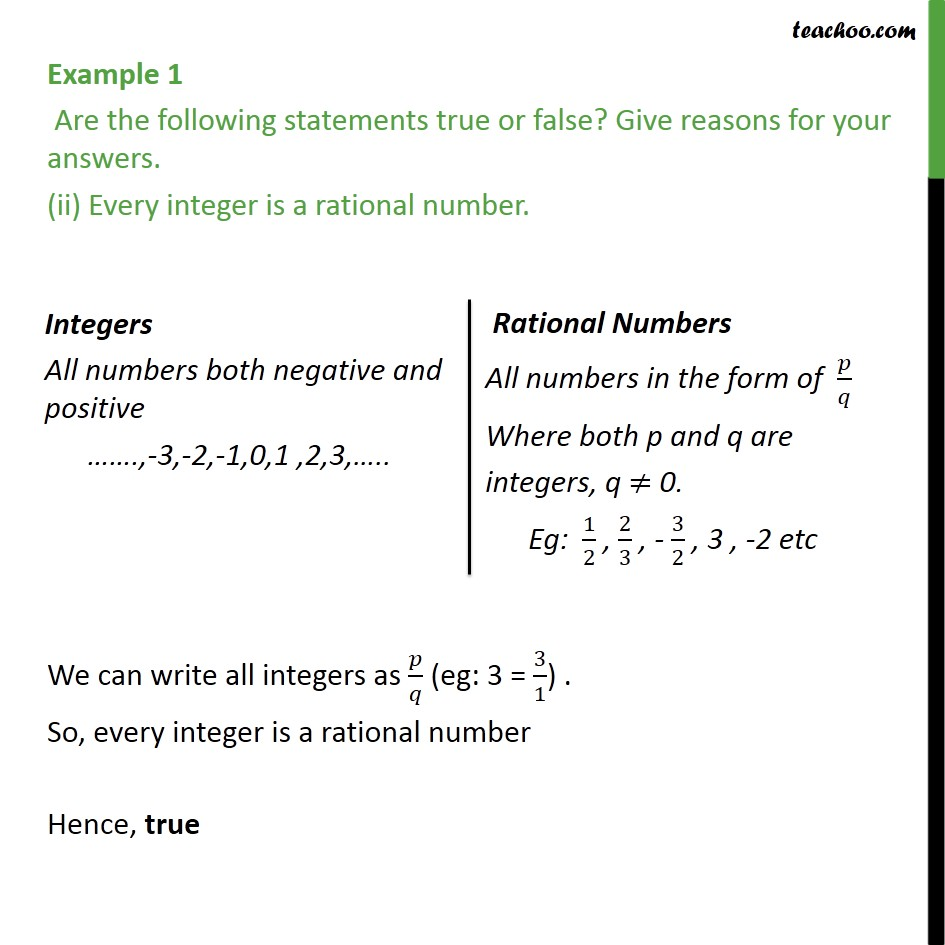Examples

Chapter 1 Class 9 Number Systems
Serial order wiseLearn in your speed, with individual attention - Teachoo Maths 1-on-1 Class

### Transcript

Example 1 Are the following statements true or false? Give reasons for your answers. (ii) Every integer is a rational number. We can write all integers as / (eg: 3 = 3/1) . So, every integer is a rational number Hence, true